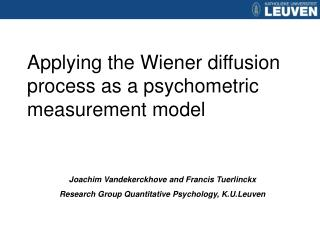DownloadDownload PresentationApplying the Wiener diffusion process as a psychometric measurement model

# Applying the Wiener diffusion process as a psychometric measurement model

Download Presentation## Applying the Wiener diffusion process as a psychometric measurement model

- - - - - - - - - - - - - - - - - - - - - - - - - - - E N D - - - - - - - - - - - - - - - - - - - - - - - - - - -
##### Presentation Transcript

1. Applying the Wiener diffusion process as a psychometric measurement model Joachim Vandekerckhove and Francis Tuerlinckx Research Group Quantitative Psychology, K.U.Leuven

2. Overview • An example problem • The diffusion model • Cognitive psychometrics • Random effects diffusion models • Explanatory diffusion models • Conclusions

3. An example problem • Speeded category verification task • Participants evaluate item category memberships

4. An example problem • Speeded category verification task • Participants evaluate item category memberships

5. An example problem • Speeded category verification task • Participants evaluate item category memberships

6. An example problem • Speeded category verification task • Participants evaluate item category memberships

7. An example problem • Speeded category verification task • Participants evaluate item category memberships

8. An example problem • Speeded category verification task • Participants evaluate item category memberships • Measure both RT and response

9. An example problem • Speeded category verification task • Participants evaluate item category memberships • Measure both RT and response • Each participant evaluates each item exactly once • Expectation: “Typical” members (e.g., Dog, Cat) are easier

10. An example problem • The problem • Standard assumptions violated • Bivariate data (RT and binary response) do not conform to the assumptions made by standard models (e.g., normality) • Different sources of variability • RT and response are partly determined by both participants (ability) and items (difficulty)

11. An example problem • The problem • Standard assumptions violated • Typical problem in mathematical psychology • Approach: use process models

12. An example problem • The problem • Standard assumptions violated • Typical problem in mathematical psychology • Approach: use process models • Different sources of variability • Typical problem in psychometrics • Approach: hierarchical models (multilevel models; mixed models; e.g., crossed random effects of persons and items)

13. Wiener diffusion model Process model for choice RT Predicts RT and binary choice simultaneously Principle: Accumulation of information Diffusion model

14. τ d Evidence a z = ba 0.0 0.125 0.250 0.375 0.500 0.625 0.750 (For persons p, conditions i, and trials j.) time

15. Diffusion model • Many associated problems • Technical issues • Parameter estimation / Model comparison • Substantive issues • Difficult to combine information across participants • Problem if many participants with few data each • Problem if items are presented only once (e.g., words) • Unlikely that parameters are constant in time (i.e., unexplained variability) • Almost completely descriptive – differences over persons/trials/conditions cannot be explained

16. Cognitive psychometrics • Use cognitive models as measurement model • Try to explain differences • between trials, manipulations and persons • by regressing the parameters on covariates

17. Indexes p for persons, i for conditions Cognitive psychometrics • Most common measurement model: Gaussian • Normal linear model (linear regression, ANOVA): • But often not a realistic model • Unsuited for choice RT

18. Measurement level describes the data Regression component explains differences Transform the parameter(s) to a linear scale Cognitive psychometrics • Common measurement model in psychometrics: Logistic • Two-parameter logistic model (item response theory):

19. Adding random effects • Not all data points come from the same distribution • Differences between participants/items/… exist, but causes unknown

20. Adding random effects • Case of the diffusion model’s drift rate

21. Ratcliff diffusion model Measurement level (Wiener process) Trial-to-trial variability in bias Trial-to-trial variability in information uptake rate Trial-to-trial variability in nondecision time Adding random effects

22. Adding random effects • Crossed random effects diffusion model

23. Adding random effects • Addition of random effects • Allows for excess variability • Due to item differences • Due to person differences • Allows to build “levels of randomness” • Importantly, can be accomplished with the diffusion model • Only feasible in a Bayesian statistical framework

24. Applying to data • Crossed random effects diffusion model Pop. distr. of Mean Stdev item easiness (distractors) item easiness (targets) person aptitude

25. Explanatory modeling • Previous models were descriptive • Didn’t use covariates • Mixed models merely quantify variability • Use external factors as predictors to • analyze the data • explain the differences in parameter values (i.e., reduce unexplained variance)

26. Explanatory modeling • Variability in choice RT due to • Inherent (stochastic) variability in sampling • Trial-to-trial differences • Participant effects • Participant’s group membership • Item effects • Item type • Combination of the above • …

27. Explanatory modeling • Use basic “building blocks” for modeling • Random/Fixed effects • Person/Item side • Hierarchical/Crossed • Use covariates (continuous/categorical/binary)

28. explaining variability in drift rate Explanatory modeling

29. Applying to data

30. Conclusions • Category verification data • Variance in person aptitude small (0.04) relative to variance in item easiness (≈ 0.11) • Item easiness correlates with typicality

31. Conclusions • More results (not discussed) • Other parameters besides drift rate may be analyzed • e.g., encoding time is negatively correlated with word length (at ±7ms/letter) • Results hold across semantic categories (not just for mammals)

32. General conclusion • Hierarchical diffusion models • combine a realistic process model for choice and reaction time with random effects and explanatory covariates • allow to analyze complex data sets in a statistically (and substantively) principled fashion with relative ease

33. Future work • Efficient software for fitting hierarchical diffusion models • Model selection and evaluation methods

34. Thank you • Questions, comments, suggestions welcome